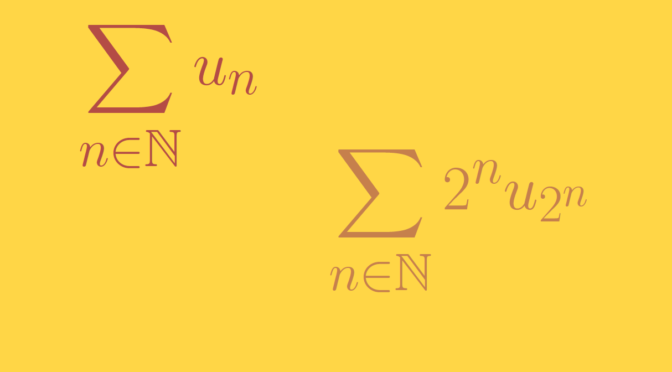# Counterexamples around Cauchy condensation test

According to Cauchy condensation test: for a non-negative, non-increasing sequence $$(u_n)_{n \in \mathbb N}$$ of real numbers, the series $$\sum_{n \in \mathbb N} u_n$$ converges if and only if the condensed series $$\sum_{n \in \mathbb N} 2^n u_{2^n}$$ converges.

The test doesn’t hold for any non-negative sequence. Let’s have a look at counterexamples.

### A sequence such that $$\sum_{n \in \mathbb N} u_n$$ converges and $$\sum_{n \in \mathbb N} 2^n u_{2^n}$$ diverges

Consider the sequence $u_n=\begin{cases} \frac{1}{n} & \text{ for } n \in \{2^k \ ; \ k \in \mathbb N\}\\ 0 & \text{ else} \end{cases}$ For $$n \in \mathbb N$$ we have $0 \le \sum_{k = 1}^n u_k \le \sum_{k = 1}^{2^n} u_k = \sum_{k = 1}^{n} \frac{1}{2^k} < 1,$ therefore $$\sum_{n \in \mathbb N} u_n$$ converges as its partial sums are positive and bounded above. However $\sum_{k=1}^n 2^k u_{2^k} = \sum_{k=1}^n 1 = n,$ so $$\sum_{n \in \mathbb N} 2^n u_{2^n}$$ diverges.

### A sequence such that $$\sum_{n \in \mathbb N} v_n$$ diverges and $$\sum_{n \in \mathbb N} 2^n v_{2^n}$$ converges

Consider the sequence $v_n=\begin{cases} 0 & \text{ for } n \in \{2^k \ ; \ k \in \mathbb N\}\\ \frac{1}{n} & \text{ else} \end{cases}$ We have $\sum_{k = 1}^{2^n} v_k = \sum_{k = 1}^{2^n} \frac{1}{k} – \sum_{k = 1}^{n} \frac{1}{2^k} > \sum_{k = 1}^{2^n} \frac{1}{k} -1$ which proves that the series $$\sum_{n \in \mathbb N} v_n$$ diverges as the harmonic series is divergent. However for $$n \in \mathbb N$$, $$2^n v_{2^n} = 0$$ and $$\sum_{n \in \mathbb N} 2^n v_{2^n}$$ converges.